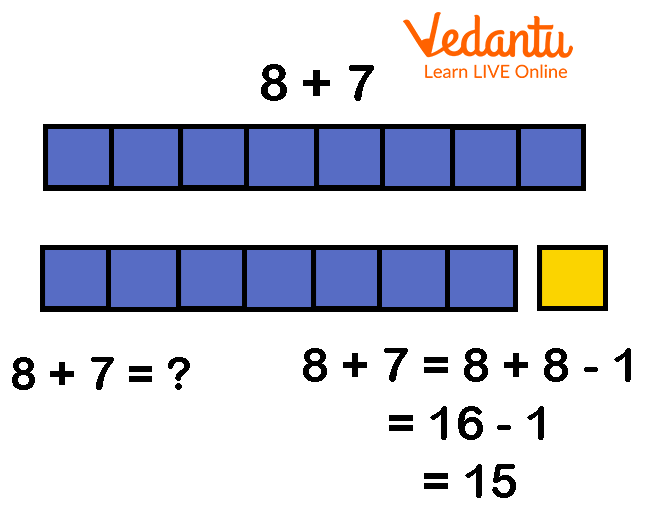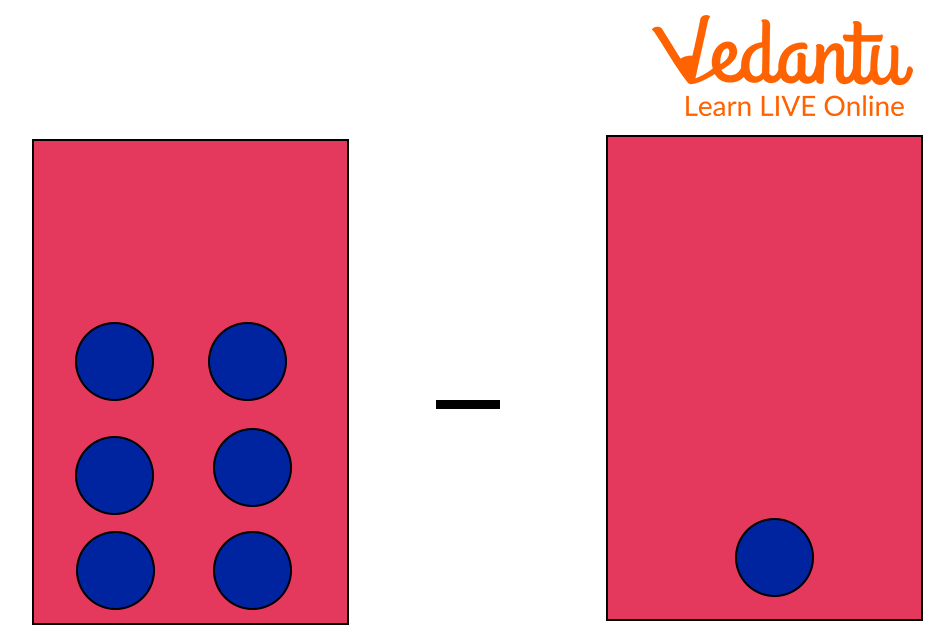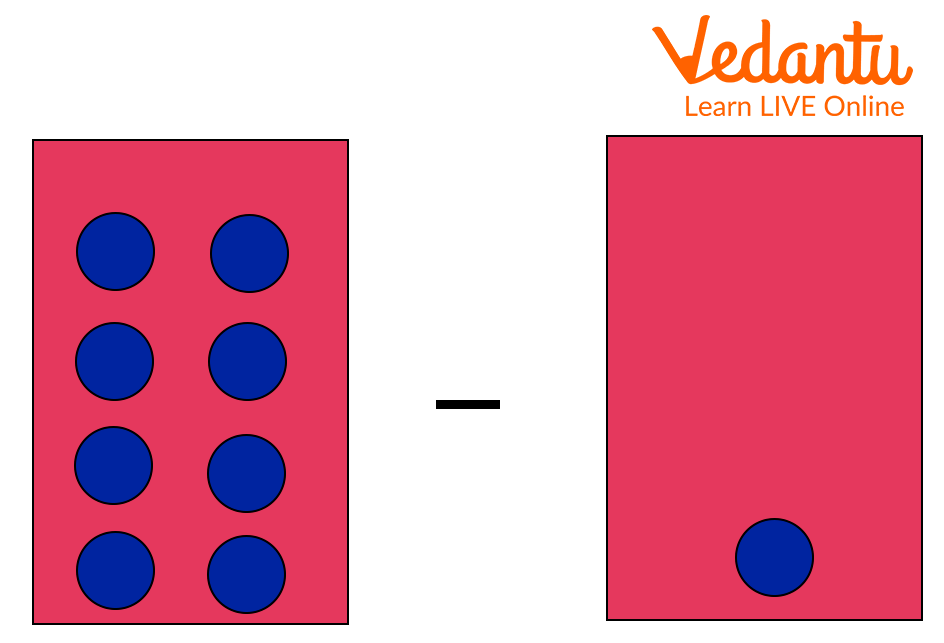Courses
Courses for Kids
Free study material
Free LIVE classes
More

# Double Minus One Facts## What does Double Minus One Fact State?

Double Minus One Fact is a strategy used to add two consecutive numbers (numbers that are next to each other). In this strategy, you simply add a bigger number to itself or double it and subtract 1 to get the final result. Let us understand double minus one fact with an example.

Look at the example below. Here, two consecutive numbers, i.e., 7 and 8 have been added using the double minus one strategy.Double Minus One Fact 3

In the above example, we have doubled the bigger number, i.e., 8 and subtracted 1 to get the final answer, i.e., 15.

Below are a few more examples of the double minus one strategy.

Example 1:4, 3 Apples

What would be 4 + 3 using the double minus strategy?

Let’s now apply the double minus strategy.Double Minus One Fact

4 + 3 = ? 4 + 3 = 4 + 4 - 1 =

7 = 8 - 1

= 7

In the above example, we have doubled the bigger number, i.e., 4 and subtracted 1 to get the final answer, i.e., 7.

Example: 23, 2 Teddy

What would be 3 + 2 using the double minus strategy?

Let’s now apply the double minus strategy.Double Minus One Fact

3 + 2 = ? 3 + 2 = 3 + 3 - 1 =

5 = 6 - 1

= 5

In the above example, we have doubled the bigger number, i.e., 3 and subtracted 1 to get the final answer, i.e., 5.

## Double Minus One Fact Solved Examples

Use double minus one fact and solve the following example:

1. 7  + 6 = ?

Solution:

Using the double minus one strategy, we get

7 + 6 = 7 + 7-1

= 14 - 1

= 13

Here, we have doubled the bigger number, i.e., 7 and subtracted 1 to get the final answer, i.e., 13.

Therefore, 7 + 6 = 13 using double minus one strategy.

2. 10 + 9 = ?

Solution:

Using double minus one strategy, we get

10 + 9 = 10 + 10 - 1

= 20 - 1

= 19

Here, we have doubled the bigger number, i.e., 10 and subtracted 1 to get the final answer, i.e., 19.

Therefore, 10 + 9 = 19 using the double minus one strategy.

## Do You Know?

• In Mathematics, double facts state that a double of 5 is 10 because 5 + 5 = 10. In the double fact, you just add the number to itself.

• The double minus one strategy is only applied to the consecutive numbers or numbers next to each other.

## Conclusion

In short, double minus one fact states that ‘double bigger’ and then just “subtract one”. This enables you to answer the questions of how adjacent or consecutive numbers are added using the double minus one strategy. For example, to add 7 + 8, double the bigger number, i.e., 8 and subtract 1. Accordingly, 8 + 8 - 1 = 15. Therefore, 8 + 7 = 15 using double minus one strategy.

Last updated date: 01st Oct 2023
Total views: 133.5k
Views today: 4.33k

## FAQs on Double Minus One Facts

1. What is the double minus one fact strategy?

In the double minus one fact strategy, we simply add the bigger number to itself or double it and then subtract 1 to get the final answer. For example, the numbers 4 and 5 using the double minus one fact strategy can be added as 5 + 5 - 1= 10 - 1 = 9.

2. What is the difference between double plus one fact and double minus one fact?

Although both double plus one and double minus fact is applied to the consecutive numbers, there is a difference between the two. The difference between double plus one fact and double minus one fact is that in the double plus one strategy, we simply add the smaller number to itself or double it and then add 1 to get the final answer. For example, consecutive numbers 8 and 9 using the double plus one fact strategy can be added as 8 + 8 + 1 = 17.

On the other hand, in the double minus one strategy, we simply add the bigger number to itself or double it and then subtract 1 to get the final answer. For example, consecutive numbers 8 and 9 using the double minus one fact strategy can be added as 9 + 9 - 1 = 17.